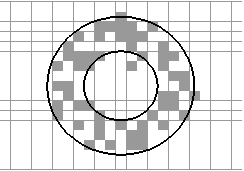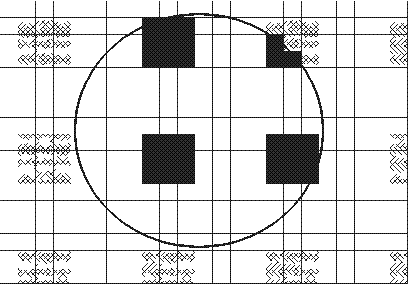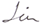LSTATS enables to calculate different statistics locally from nominal or numerical byte format raster layers (Idrisi32 rst-format), and also colour separations from RGB rasters. Local statistics are calculated within a moving window called kernel. LSTATS is working under Windows operating system. Special features Input and output layers may have different boundaries and resolution (pixel size). Some indices of spatial pattern rarely found in other applications. E.g. gradient strength, stripeness, and mode weighted by inverse distance. Extensive possibilities in the choice of local kernel and sample parameters. Local statistics can be calculated at locations given by a list of coordinates or at pixels of the output layer layer. The output of calculated indices can be directed either to an ASCII file or to a binary raster. The area of calculations can be irregular defined by void values in a mask file. LSTATS can be used to convert data from ASCII to binary, from binary to ASCII formats and between binary files of different boundaries and resolution. The square shaped, round, sector- or an annulus-kernel can be chosen (link to figures demonstrating samples and kernel options from Linder et al). All pixels or a sample of pixels from the chosen kernel can be used in calculations. The sample can be star-shaped, random (with user-defined probability of selection), or regular grid of blocks of 3 x 3 pixels (link to figures demonstrating kernel options, see below). Kernel optionsStar-shaped sample in round kernel Star-shaped sample (black squares) from a circular kernel (circle radius 5 pixels).Random sample in an annulus kernel Random 50% sample within an annulus kernel (internal radius 3.5, external radius 7 pixels) on a raster layer.Block sample in a circular kernel Block sample and a circular kernel (radius = 7 pixels) on a raster layer. Sample share 9/49 (black and cross-hatched squares). Black squares are used for calculation of local statistics in this kernel.

 Last update 28.08.2014 Webmaster